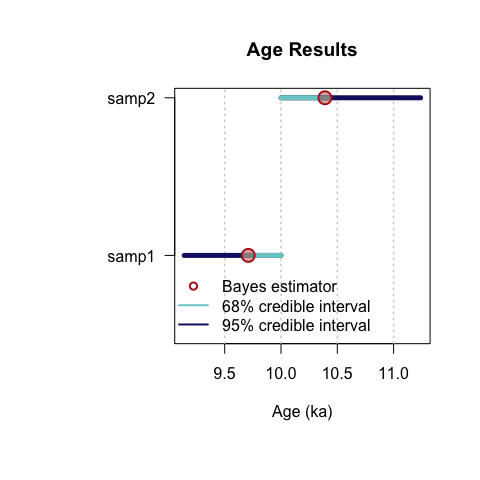# Introduction

‘BayLum’ provides a collection of various R functions for Bayesian analysis of luminescence data. Amongst others, this includes data import, export, application of age models and palaeodose modelling.

Data can be processed simultaneously for various samples, including the input of multiple BIN/BINX-files per sample for single grain (SG) or multigrain (MG) OSL measurements. Stratigraphic constraints and systematic errors can be added to constrain the analysis further.

For those who already know how to use R, ‘BayLum’ won’t be difficult to use, for all others, this brief introduction may be of help to make the first steps with R and the package ‘BayLum’ as convenient as possible.

## Installing BayLum’ package

If you read this document before having installed R itself, you should first visit the R project webpage and download and install R. You may also consider installing Rstudio, which provides an excellent desktop working environment for R; however it is not a prerequisite.

Despite, you will need the external software JAGS (Just Another Gibs Sampler). Please visit the JAGS webpage and follow the installation instructions. Now you are nearly ready to work with ‘BayLum’.

If you have not yet installed BayLum’, please run the following two R code lines to install ‘BayLum’ on your computer.

if(!require("BayLum"))
install.packages("BayLum", dependencies = TRUE)

Alternatively, you can load an already installed R package (here ‘BayLum’) into your session by using the following R call.

library(BayLum)

# First steps: age analysis of one sample

## Import information from a BIN/BINX-file.

Let us consider the sample is named samp1, which is the example dataset coming with the package. All information related to this sample is stored in a subfolder called also samp1. To test the package example, first, we add the path of the example dataset to the object path.

path <- paste0(system.file("extdata/", package = "BayLum"), "/")

Please note that for your own dataset (i.e. not included in the package) you have to replace this call by something like:

path <- "Users/Master_of_luminescence/Documents/MyFamousOSLData"

In our example the folder contains the following subfolders and files:

 1 FER1/bin.BIN 2 FER1/Disc.csv 3 FER1/DoseEnv.csv 4 FER1/DoseSource.csv 5 FER1/rule.csv 6 samp1/bin.BIN 7 samp1/DiscPos.csv 8 samp1/DoseEnv.csv 9 samp1/DoseSource.csv 10 samp1/rule.csv 11 samp2/bin.BIN 12 samp2/DiscPos.csv 13 samp2/DoseEnv.csv 14 samp2/DoseSource.csv 15 samp2/rule.csv

See “What are the required files in each subfolder?” in the manual of Generate_DataFile() function for the meaning of these files.

To import your data, simply call the function Generate_DataFile():

DATA1 <-
Generate_DataFile(
Path = path,
FolderNames = "samp1",
Nb_sample = 1,
verbose = FALSE
)

### Remarks

#### Data import/export

The import may take a while, in particular for large BIN/BINX-files. This can become annoying if you want to play with the data. In such situations, it makes sense to save your imported data somewhere else before continuing.

To save the obove imported data on your hardrive use

save(DATA1, file = "YourPath/DATA1.RData")

To load the data use

load(DATA1, file = "YourPath/DATA1.RData")

#### Data structure

To see the overall structure of the data generated from the BIN/BINX-file and the associated CSV-files, the following call can be used:

str(DATA1)
List of 9
$LT :List of 1 ..$ : num [1, 1:7] 2.042 0.842 1.678 3.826 4.258 ...
$sLT :List of 1 ..$ : num [1, 1:7] 0.344 0.162 0.328 0.803 0.941 ...
$ITimes :List of 1 ..$ : num [1, 1:6] 15 30 60 100 0 15
$dLab : num [1:2, 1] 1.53e-01 5.89e-05$ ddot_env      : num [1:2, 1] 2.512 0.0563
$regDose :List of 1 ..$ : num [1, 1:6] 2.3 4.6 9.21 15.35 0 ...
$J : num 1$ K             : num 6
$Nb_measurement: num 16 It reveals that DATA1 is basically a list with 9 elements: Element Content DATA1$LT Lx/Tx values from each sample
DATA1$sLT Lx/Tx error values from each sample DATA1$ITimes Irradiation times
DATA1$dLab The lab dose rate DATA1$ddot_env The environmental dose rate and its variance
DATA1$regDose The regenarated dose points DATA1$J The number of aliquots selected for each BIN-file
DATA1$K The number of regenarted dose points DATA1$Nb_measurement The number of measurements per BIN-file

#### Visualise Lx/Tx values and dose points

To get an impression on how your data look like, you can visualise them by using the function LT_RegenDose():

LT_RegenDose(
DATA = DATA1,
Path = path,
FolderNames = "samp1",
SampleNames = "samp1",
Nb_sample = 1,
nrow = NULL
)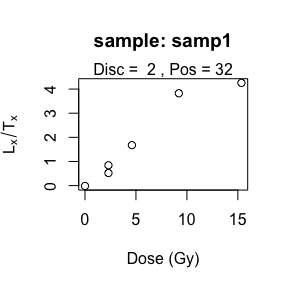Note that here we consider only one sample, and the name of the folder is the name of the sample. For that reason the argumetns were set to FolderNames = samp1 and SampleNames = samp1.

## Generate data file from BIN/BINX-files of multi-grain OSL measurements

For a multi-grain OSL measurements, instead of Generate_DataFile(), the function Generate_DataFile_MG() should be used with similar parameters. The functions differ by their expectations: Disc.csv instead of DiscPos.csv file for Single-grain OSL Measurements. Please check type ?Generate_DataFile_MG for further information.

## Age computation

To compute the age of the sample samp1, you can run the following code:

Age <- Age_Computation(
DATA = DATA1,
SampleName = "samp1",
PriorAge = c(10, 100),
distribution = "cauchy",
LIN_fit = TRUE,
Origin_fit = FALSE,
Iter = 10000
)
Compiling model graph
Resolving undeclared variables
Allocating nodes
Graph information:
Observed stochastic nodes: 6
Unobserved stochastic nodes: 9
Total graph size: 139

Initializing model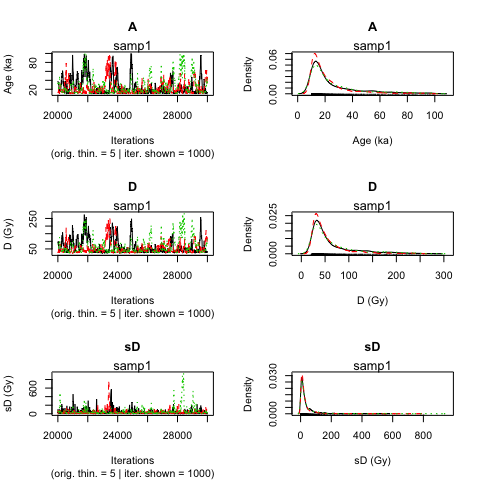>> Sample name <<
----------------------------------------------
samp1

>> Results of the Gelman and Rubin criterion of convergence <<
----------------------------------------------
Point estimate Uppers confidence interval
A    1.02        1.05
D    1.02        1.06
sD   1.08        1.12

---------------------------------------------------------------------------------------------------
*** WARNING: The following information are only valid if the MCMC chains have converged  ***
---------------------------------------------------------------------------------------------------

parameter    Bayes estimate       Credible interval
----------------------------------------------
A        24.373
lower bound     upper bound
at level 95%    10          67.99
at level 68%    10          24.49
----------------------------------------------
D        60.659
lower bound     upper bound
at level 95%    20.37       169.95
at level 68%    22.02       61.34
----------------------------------------------
sD       39.715
lower bound     upper bound
at level 95%    0.03        138.27
at level 68%    0.31        30.22 

This also works if DATA1 is the output of Generate_DataFile_MG().

##### Remark 1: MCMC trajectories
• If MCMC trajectories did not converge, you can add more iteration with the parameter Iter in the function Age_Computation(), for example Iter = 20000 or Iter = 50000.

• To increase the precision of prior distribution, if not specified before you can use the argument PriorAge. For example: PriorAge= c(0.01,10) for a young sample and PriorAge = c(10,100) for an old sample.

• If the trajectories are still not convergering, you should whether the choice you made with the argument distribution and dose-response curves are meaningful.

##### Remark 2: LIN_fit and Origin_fit, dose-response curves option
• By default, a saturating exponential plus linear dose response curve is expected. However, you choose other formula by changing arguments LIN_fit and Origin_fit in the function.
##### Remark 3: distribution, equivalent dose dispersion option

By default, a cauchy distribution is assumed, but you can choose another distribution by replacing the word cauchy by gaussian, lognormal_A or lognormal_M for the argument distribution.

The difference between the models: lognormal_A and lognormal_M is that the equivalent dose dispersion are distributed according to:

• a lognormal distribution with mean or average equal to the palaeodose for the first model
• a lognormal distribution with median equal to the palaeodose for the second model.
##### Remark 4: SavePdf and SaveEstimates option

These two arguments allow to save the results to files.

• SavePdf = TRUE create a PDF-file with MCMC trajectories of parameters A (age), D (palaeodose), sD (equivalent doses dispersion). You have to specify OutputFileName and OutputFilePath to define name and path of the PDF-file.

• SaveEstimates = TRUE saves a CSV-file containing the Bayes estimates, the credible interval at 68% and 95% and the Gelman and Rudin test of convergency of the parameters A, D, sD. For the export the arugments OutputTableName and OutputTablePath have to be specified.

##### Remark 4: PriorAge option

By default, an age between 0.01 ka and 100 ka is expected. If the user has more informations on the sample, PriorAge should be modified accordingly.

For example, if you know that the sample is an older, you can set PriorAge=c(10,120). In contrast, if you know that the sample is younger, you may want to set PriorAge=c(0.001,10). Ages of $$<=0$$ are not possible. The minimum bound is 0.001.

Please note that the setting of PriorAge is not trivial, wrongly set boundaries are likely biasing your results.

## Multiple BIN/BINX-files for one sample

In the previous example we considered only the simplest case: one sample, and one BIN/BINX-file. However, ‘BayLum’ allows to process multiple BIN/BINX-files for one sample. To work with multiple BIN/BINX-files, the names of the subfolders need to beset in argument Names and both files need to be located unter the same Path.

For the case

Names <- c("samp1", "samp2")

the call Generate_DataFile() (or Generate_DataFile_MG()) becomes as follows:

##argument setting
nbsample <- 1
nbbinfile <- length(Names)
Binpersample <- c(length(Names))

##call data file generator
DATA_BF <- Generate_DataFile(
Path = path,
FolderNames = Names,
Nb_sample = nbsample,
Nb_binfile = nbbinfile,
BinPerSample = Binpersample,
verbose = FALSE
)

##calculate the age
Age <- Age_Computation(
DATA = DATA_BF,
SampleName = Names,
BinPerSample = Binpersample
)
Compiling model graph
Resolving undeclared variables
Allocating nodes
Graph information:
Observed stochastic nodes: 12
Unobserved stochastic nodes: 15
Total graph size: 221

Initializing model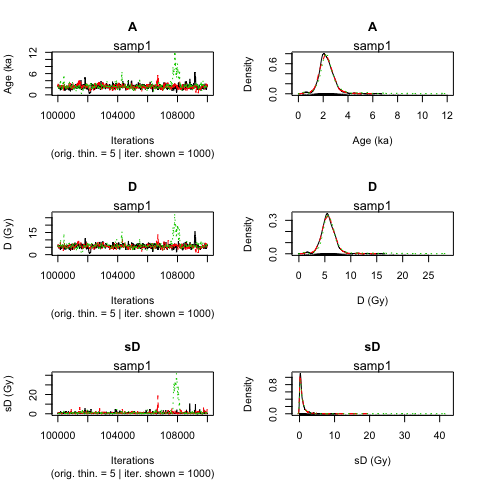>> Sample name <<
----------------------------------------------
samp1 samp2

>> Results of the Gelman and Rubin criterion of convergence <<
----------------------------------------------
Point estimate Uppers confidence interval
A    1.13        1.26
D    1.14        1.29
sD   1.27        1.75

---------------------------------------------------------------------------------------------------
*** WARNING: The following information are only valid if the MCMC chains have converged  ***
---------------------------------------------------------------------------------------------------

parameter    Bayes estimate       Credible interval
----------------------------------------------
A        2.364
lower bound     upper bound
at level 95%    1.08        3.38
at level 68%    1.71        2.7
----------------------------------------------
D        5.876
lower bound     upper bound
at level 95%    2.88        8.18
at level 68%    4.39        6.7
----------------------------------------------
sD       1.123
lower bound     upper bound
at level 95%    0       2.43
at level 68%    0       0.75 

# Age analysis of various samples

## Generate data file from BIN/BINX-files

The function Generate_DataFile() (or Generate_DataFile_MF()) can process multiple files simultaneously including multiple BIN/BINX-files per sample.

We assume that we are interested in two samples named: sample1 and sample2. In addition, we have two BIN/BINX-files for the first sample named: sample1-1 and sample1-2, and one BIN-file for the 2nd sample named sample2-1. In such case, we need three subfolders named sample1-1, sample1-2 and sample2-1; which each subfolder containing only one BIN-file named bin.BIN, and its associated files DiscPos.csv, DoseEnv.csv, DoseSourve.csv and rule.csv. All of these 3 subfolders must be located in path.

To fill the argument corectly BinPerSample: $$binpersample=c(\underbrace{2}_{\text{sample 1: 2 bin files}},\underbrace{1}_{\text{sample 2: 1 bin file}})$$

Names <-
c("sample1-1", "sample1-2", "sample2-1") # give the name of the folder datat
nbsample <- 2    # give the number of samples
nbbinfile <- 3   # give the number of bin files
DATA <- Generate_DataFile(
Path = path,
FolderNames = Names,
Nb_sample = nbsample,
Nb_binfile = nbbinfile,
BinPerSample = binpersample
)

### Combine files using the function combine_DataFiles()

If the user has already saved informations imported with Generate_DataFile() function (or Generate_DataFile_MG() function) these data can be concatenate with the function combine_DataFiles().

For example, if DATA1 is the output of sample named “GDB3”, and DATA2 is the output of sample “GDB5”, both data can be merged with the following call:

data("DATA1", envir = environment())
data("DATA2", envir = environment())
DATA3 <- combine_DataFiles(L1 = DATA2, L2 = DATA1)
str(DATA3)
List of 9
$LT :List of 2 ..$ : num [1:188, 1:6] 4.54 2.73 2.54 2.27 1.48 ...
..$: num [1:101, 1:6] 5.66 6.9 4.05 3.43 4.97 ...$ sLT           :List of 2
..$: num [1:188, 1:6] 0.333 0.386 0.128 0.171 0.145 ... ..$ : num [1:101, 1:6] 0.373 0.315 0.245 0.181 0.246 ...
$ITimes :List of 2 ..$ : num [1:188, 1:5] 40 40 40 40 40 40 40 40 40 40 ...
..$: num [1:101, 1:5] 160 160 160 160 160 160 160 160 160 160 ...$ dLab          : num [1:2, 1:2] 1.53e-01 5.89e-05 1.53e-01 5.89e-05
$ddot_env : num [1:2, 1:2] 2.512 0.0563 2.26 0.0617$ regDose       :List of 2
..$: num [1:188, 1:5] 6.14 6.14 6.14 6.14 6.14 6.14 6.14 6.14 6.14 6.14 ... ..$ : num [1:101, 1:5] 24.6 24.6 24.6 24.6 24.6 ...
$J : num [1:2] 188 101$ K             : num [1:2] 5 5
$Nb_measurement: num [1:2] 14 14 The data structure should become as follows • 2 lists (1 list per sample) for DATA$LT, DATA$sLT, DATA1$ITimes and DATA1$regDose • A matrix with 2 columns (1 line per sample) for DATA1$dLab, DATA1$ddot_env • 2 integers (1 integer per BIN files here we have 1 BIN-file per sample) for DATA1$J, DATA1$K, DATA1$Nb_measurement.

Single-grain and multiple-grain OSL measurements can be merged in the same way. To plot the $$L/T$$ as a function of the regenerative dose the function LT_RegenDose() can be used again:

LT_RegenDose(
DATA = DATA3,
Path = path,
FolderNames = Names,
Nb_sample = nbsample,
SG = rep(TRUE, nbsample)
)

Note: In the example DATA3 contains information from the samples ‘GDB3’ and ‘GDB5’, which are single-grain OSL measurements. For a correct treatment the argument SG has to be manually set be the user. Please see the function manual for further details.

## Age analysis without stratigraphic constraints

If there no stratigraphic constraints were set, the following code to analyse simultaneously the age of the sample GDB5 and GDB3.

priorage = c(1, 10, 10, 100)
Age <- AgeS_Computation(
DATA = DATA3,
Nb_sample = 2,
SampleNames = c("GDB5", "GDB3"),
PriorAge = priorage,
distribution = "cauchy",
LIN_fit = TRUE,
Origin_fit = FALSE,
Iter = 1000
)
Compiling model graph
Resolving undeclared variables
Allocating nodes
Graph information:
Observed stochastic nodes: 1445
Unobserved stochastic nodes: 1739
Total graph size: 20624

Initializing model
Warning: [plot_MCMC()] 'n.iter' out of range, reset to number of observations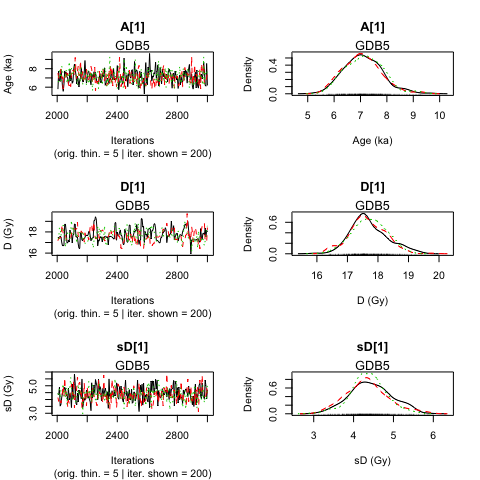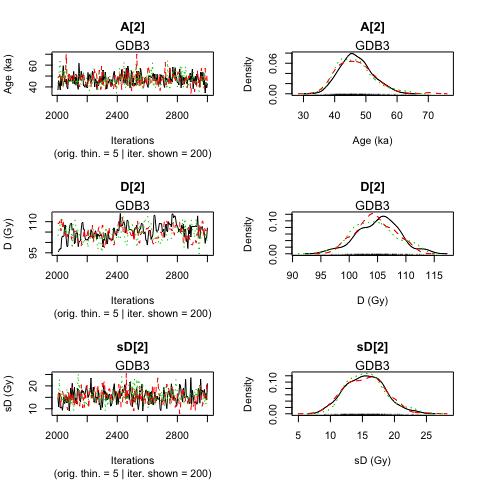>> Results of the Gelman and Rubin criterion of convergence <<
----------------------------------------------
Sample name:  GDB5
---------------------
Point estimate Uppers confidence interval
A_GDB5   1       1.01
D_GDB5   1       1.01
sD_GDB5      1.01        1.03
----------------------------------------------
Sample name:  GDB3
---------------------
Point estimate Uppers confidence interval
A_GDB3   1       1
D_GDB3   1.01        1.03
sD_GDB3      1       1

---------------------------------------------------------------------------------------------------
*** WARNING: The following information are only valid if the MCMC chains have converged  ***
---------------------------------------------------------------------------------------------------

>> Bayes estimates of Age, Palaeodose and its dispersion for each sample and credible interval <<
----------------------------------------------
Sample name:  GDB5
---------------------
Parameter    Bayes estimate       Credible interval
A_GDB5      7.108
lower bound     upper bound
at level 95%    5.71        8.35
at level 68%    6.36        7.76

Parameter    Bayes estimate       Credible interval
D_GDB5      17.696
lower bound     upper bound
at level 95%    16.6        18.9
at level 68%    17.22       18.31

Parameter    Bayes estimate       Credible interval
sD_GDB5      4.384
lower bound     upper bound
at level 95%    3.52        5.46
at level 68%    3.95        4.94
----------------------------------------------
Sample name:  GDB3
---------------------
Parameter    Bayes estimate       Credible interval
A_GDB3      46.728
lower bound     upper bound
at level 95%    37.06       57.57
at level 68%    39.86       50.04

Parameter    Bayes estimate       Credible interval
D_GDB3      104.739
lower bound     upper bound
at level 95%    98.5        112.54
at level 68%    101         108.03

Parameter    Bayes estimate       Credible interval
sD_GDB3      15.522
lower bound     upper bound
at level 95%    9.68        21.13
at level 68%    11.94       17.73

----------------------------------------------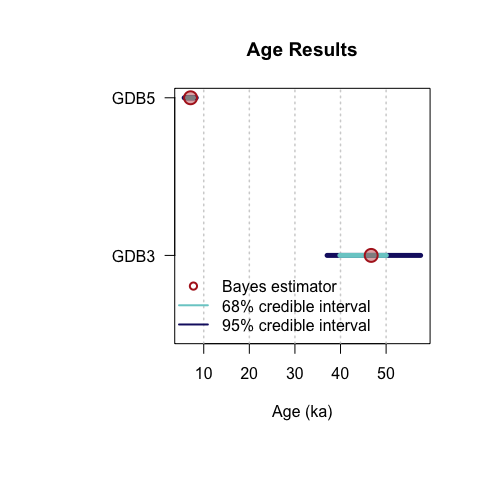### Remarks

If the MCMC trajectories did not converge, we refer to the comments above.

As for the function Age_computation(), the age for each sample is set by default between 0.01 ka and 100 ka. If you have more informations on your samples it is possible to change PriorAge parameters. PriorAge is a vector of size = 2*$Nb_sample, the two first values of PriorAge concern the 1st sample, the next two values the 2nd sample and so on. For example, if you know that sample named GDB5 is a young sample whose its age is between 0.01 ka and 10 ka, and GDB3 is an old sample whose age is between 10 ka and 100 ka, $PriorAge=c(\underbrace{0.01,10}_{GDB5\ prior\ age},\underbrace{10,100}_{GDB3\ prior\ age})$ ## Age analysis with stratigraphic constraints With the function AgeS_Computation() it is possible to take the stratigraphic relations between samples into account and define constraints. For example, we know that GDB5 is in a higher stratigraphical position, hence it likely has a younger age than sample GDB3. ### Ordering samples To take into account stratigraphic constraints, the information on the samples need to be ordered. Either you enter a sample name (corresponding to subfolder names) in Names parameter of the function Generate_DataFile(), ordered by order of increasing ages or you enter saved .RData informations of each sample in combine_DataFiles(), ordered by increasing ages. # using Generate_DataFile function Names <- c("samp1", "samp2") nbsample <- 2 DATA3 <- Generate_DataFile( Path = path, FolderNames = Names, Nb_sample = nbsample, verbose = FALSE ) # using the function combine_DataFiles() data(DATA1, envir = environment()) # .RData on sample GDB3 data(DATA2, envir = environment()) # .RData on sample GDB5 DATA3 <- combine_DataFiles(L1 = DATA1, L2 = DATA2) ### Define matrix to set stratigraphic constraints Let SC be the matrix containing all information on stratigraphic relations for this two samples. This matrix is defined as follows: • matrix dimensions: the row number of StratiConstraints matrix is equal to Nb_sample+1, and column number is equal to $$Nb\_sample$$. • first matrix row: for all $$i$$ in $$\{1,...,Nb\_Sample\}$$, StratiConstraints[1,i] <- 1, means that the lower bound of the sample age given in PriorAge[2i-1] for the sample whose number ID is equal to $$i$$ is taken into account • sample relations: for all $$j$$ in${2,…,Nb_Sample+1}\$ and all $$i$$ in $$\{j,...,Nb\_Sample\}$$, StratiConstraints[j,i] <- 1 if the sample age whose ID is equal to $$j-1$$ is lower than the sample age whose ID is equal to $$i$$. Otherwise, StratiConstraints[j,i] <- 0.

To the define such matrix the function SCMatrix() can be used:

SC <- SCMatrix(Nb_sample = 2,
SampleNames = c("samp1", "samp2"))

In our case: 2 samples, SC is a matrix with 3 rows and 2 columns. The first row contains c(1,1) (because we take into account the prior ages), the second line contains c(0,1) (sample 2, named samp2 is supposed to be older than sample 1, named samp1) and the third line contains c(0,0) (sample 2, named samp2 is not younger than the sample 1, here named samp1). We can also fill the matrix with the stratigraphic relations as follow:

SC <- matrix(
data = c(1, 1, 0, 1, 0, 0),
ncol = 2,
nrow = (2 + 1),
byrow = T
)

### Age computation

Age <-
AgeS_Computation(
DATA = DATA3,
Nb_sample = 2,
SampleNames = c("samp1", "samp2"),
PriorAge = priorage,
distribution = "cauchy",
LIN_fit = TRUE,
Origin_fit = FALSE,
StratiConstraints = SC,
Iter = 1000
)
Compiling model graph
Resolving undeclared variables
Allocating nodes
Graph information:
Observed stochastic nodes: 1445
Unobserved stochastic nodes: 1739
Total graph size: 20624

Initializing model
Warning: [plot_MCMC()] 'n.iter' out of range, reset to number of observations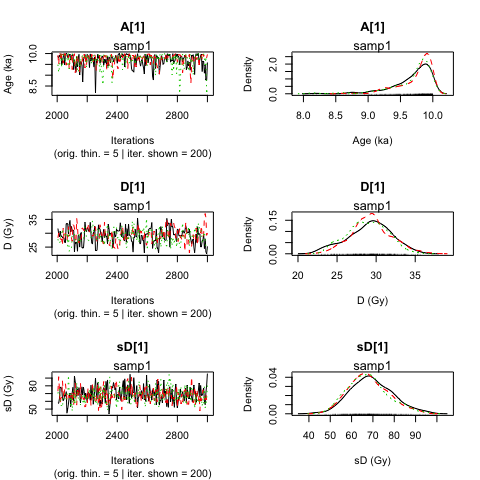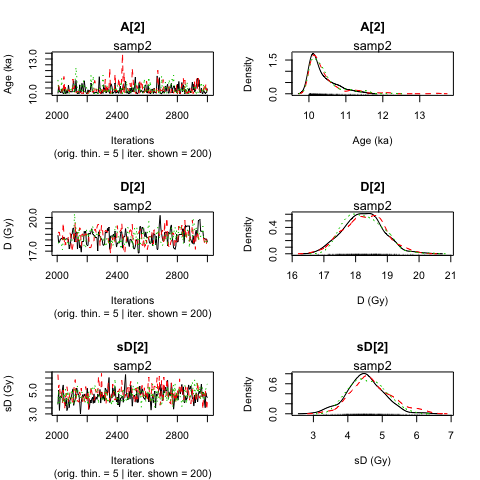>> Results of the Gelman and Rubin criterion of convergence <<
----------------------------------------------
Sample name:  samp1
---------------------
Point estimate Uppers confidence interval
A_samp1      1.02        1.04
D_samp1      1.01        1.03
sD_samp1     1       1.02
----------------------------------------------
Sample name:  samp2
---------------------
Point estimate Uppers confidence interval
A_samp2      1.02        1.03
D_samp2      1       1
sD_samp2     1.03        1.09

---------------------------------------------------------------------------------------------------
*** WARNING: The following information are only valid if the MCMC chains have converged  ***
---------------------------------------------------------------------------------------------------

>> Bayes estimates of Age, Palaeodose and its dispersion for each sample and credible interval <<
----------------------------------------------
Sample name:  samp1
---------------------
Parameter    Bayes estimate       Credible interval
A_samp1     9.709
lower bound     upper bound
at level 95%    9.14        10
at level 68%    9.67        10

Parameter    Bayes estimate       Credible interval
D_samp1     29.14
lower bound     upper bound
at level 95%    24.15       33.88
at level 68%    27.04       31.98

Parameter    Bayes estimate       Credible interval
sD_samp1     68.34
lower bound     upper bound
at level 95%    51.4        87.58
at level 68%    59.34       78
----------------------------------------------
Sample name:  samp2
---------------------
Parameter    Bayes estimate       Credible interval
A_samp2     10.39
lower bound     upper bound
at level 95%    10          11.24
at level 68%    10          10.43

Parameter    Bayes estimate       Credible interval
D_samp2     18.26
lower bound     upper bound
at level 95%    17.11       19.3
at level 68%    17.56       18.78

Parameter    Bayes estimate       Credible interval
sD_samp2     4.595
lower bound     upper bound
at level 95%    3.49        5.5
at level 68%    3.96        4.92

----------------------------------------------